Chapter 5. Stoichiometry and the Mole

# Stoichiometry

### Learning Objectives

1. Define stoichiometry.
2. Relate quantities in a balanced chemical reaction on a molecular basis.

Consider a classic recipe for pound cake: 1 pound of eggs, 1 pound of butter, 1 pound of flour, and 1 pound of sugar. (That’s why it’s called “pound cake.”) If you have 4 pounds of butter, how many pounds of sugar, flour, and eggs do you need? You would need 4 pounds each of sugar, flour, and eggs.

Now suppose you have 1.00 g H2. If the chemical reaction follows the balanced chemical equation

2 H2(g) + O2(g) → 2 H2O(ℓ)

then what mass of oxygen do you need to make water?

Curiously, this chemical reaction question is very similar to the pound cake question. Both of them involve relating a quantity of one substance to a quantity of another substance or substances. The relating of one chemical substance to another using a balanced chemical reaction is called stoichiometry. Using stoichiometry is a fundamental skill in chemistry; it greatly broadens your ability to predict what will occur and, more importantly, how much is produced.

Let us consider a more complicated example. A recipe for pancakes calls for 2 cups (c) of pancake mix, 1 egg, and 1/2 c of milk. We can write this in the form of a chemical equation:

2 c mix + 1 egg + 1/2 c milk → 1 batch of pancakes

If you have 9 c of pancake mix, how many eggs and how much milk do you need? It might take a little bit of work, but eventually you will find you need 4½ eggs and 2¼ c milk.

How can we formalize this? We can make a conversion factor using our original recipe and use that conversion factor to convert from a quantity of one substance to a quantity of another substance, similar to the way we constructed a conversion factor between feet and yards in Chapter 2 “Measurements”. Because one recipe’s worth of pancakes requires 2 c of pancake mix, 1 egg, and 1/2 c of milk, we actually have the following mathematical relationships that relate these quantities:

2 c pancake mix ⇔ 1 egg ⇔ 1/2 c milk

where ⇔ is the mathematical symbol for “is equivalent to.” This does not mean that 2 c of pancake mix equal 1 egg. However, as far as this recipe is concerned, these are the equivalent quantities needed for a single recipe of pancakes. So, any possible quantities of two or more ingredients must have the same numerical ratio as the ratios in the equivalence.

We can deal with these equivalences in the same way we deal with equalities in unit conversions: we can make conversion factors that essentially equal 1. For example, to determine how many eggs we need for 9 c of pancake mix, we construct the conversion factor: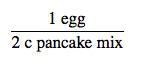This conversion factor is, in a strange way, equivalent to 1 because the recipe relates the two quantities. Starting with our initial quantity and multiplying by our conversion factor,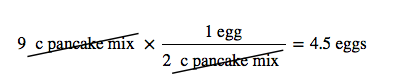Note how the units cups pancake mix canceled, leaving us with units of eggs. This is the formal, mathematical way of getting our amounts to mix with 9 c of pancake mix. We can use a similar conversion factor for the amount of milk: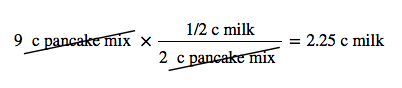Again, units cancel, and new units are introduced.

A balanced chemical equation is nothing more than a recipe for a chemical reaction. The difference is that a balanced chemical equation is written in terms of atoms and molecules, not cups, pounds, and eggs.

For example, consider the following chemical equation:

2 H2(g) + O2(g) → 2 H2O(ℓ)

We can interpret this as, literally, “two hydrogen molecules react with one oxygen molecule to make two water molecules.” That interpretation leads us directly to some equivalences, just as our pancake recipe did:

2 H2 molecules ⇔ 1 O2 molecule ⇔ 2 H2O molecules

These equivalences allow us to construct conversion factors: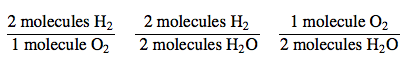and so forth. These conversions can be used to relate quantities of one substance to quantities of another. For example, suppose we need to know how many molecules of oxygen are needed to react with 16 molecules of H2. As we did with converting units, we start with our given quantity and use the appropriate conversion factor: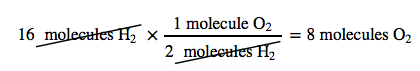Note how the unit molecules H2 cancels algebraically, just as any unit does in a conversion like this. The conversion factor came directly from the coefficients in the balanced chemical equation. This is another reason why a properly balanced chemical equation is important.

### Example 1

How many molecules of SO3 are needed to react with 144 molecules of Fe2O3 given this balanced chemical equation?

Fe2O3(s) + 3SO3(g) → Fe2(SO4)3

Solution

We use the balanced chemical equation to construct a conversion factor between Fe2O3 and SO3. The number of molecules of Fe2O3 goes on the bottom of our conversion factor so it cancels with our given amount, and the molecules of SO3 go on the top. Thus, the appropriate conversion factor is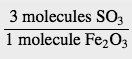Starting with our given amount and applying the conversion factor, the result is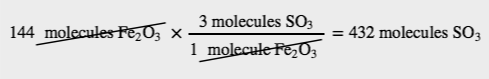We need 432 molecules of SO3 to react with 144 molecules of Fe2O3.

Test Yourself

How many molecules of H2 are needed to react with 29 molecules of N2 to make ammonia if the balanced chemical equation is N2 + 3H2 → 2NH3?

Answer

87 molecules

Chemical equations also allow us to make conversions regarding the number of atoms in a chemical reaction because a chemical formula lists the number of atoms of each element in a compound. The formula H2O indicates that there are two hydrogen atoms and one oxygen atom in each molecule, and these relationships can be used to make conversion factors: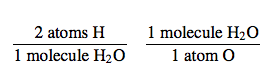Conversion factors like this can also be used in stoichiometry calculations.

### Example 2

How many molecules of NH3 can you make if you have 228 atoms of H2?

Solution

From the formula, we know that one molecule of NH3 has three H atoms. Use that fact as a conversion factor: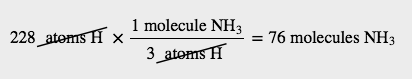Test Yourself

How many molecules of Fe2(SO4)3 can you make from 777 atoms of S?

Answer

259 moleculesVideo Source: Stiochiometry by keyj (https://viuvideos.viu.ca/media/Stoichiometry/0_b6t2h6o2)

### Key Takeaway

• Quantities of substances can be related to each other using balanced chemical equations.

### Exercises

1. Think back to the pound cake recipe. What possible conversion factors can you construct relating the components of the recipe?

2. Think back to the pancake recipe. What possible conversion factors can you construct relating the components of the recipe?

3. What are all the conversion factors that can be constructed from the balanced chemical reaction 2H2(g) + O2(g) → 2H2O(ℓ)?

4. What are all the conversion factors that can be constructed from the balanced chemical reaction N2(g) + 3H2(g) → 2NH3(g)?

5. Given the chemical equation

Na(s) + H2O(ℓ) → NaOH(aq) + H2(g)

a)  Balance the equation.

b)  How many molecules of H2 are produced when 332 atoms of Na react?

6.  Given the chemical equation

S(s) + O2(g) → SO3(g)

a)  Balance the equation.

b)  How many molecules of O2 are needed when 38 atoms of S react?

7.  For the balanced chemical equation

6 H+(aq) + 2 MnO4(aq) + 5 H2O2(ℓ) → 2 Mn2+(aq) + 5 O2(g) + 8 H2O(ℓ)

how many molecules of H2O are produced when 75 molecules of H2O2 react?

8.  For the balanced chemical reaction

2 C6H6(ℓ) + 15 O2(g) → 12 CO2(g) + 6 H2O(ℓ)

how many molecules of CO2 are produced when 56 molecules of C6H6 react?

9.  Given the balanced chemical equation

Fe2O3(s) + 3SO3(g) → Fe2(SO4)3

how many molecules of Fe2(SO4)3 are produced if 321 atoms of S are reacted?

10.  For the balanced chemical equation

CuO(s) + H2S(g) → CuS + H2O(ℓ)

how many molecules of CuS are formed if 9,044 atoms of H react?

11.  For the balanced chemical equation

Fe2O3(s) + 3 SO3(g) → Fe2(SO4)3

suppose we need to make 145,000 molecules of Fe2(SO4)3. How many molecules of SO3 do we need?

12.  One way to make sulfur hexafluoride is to react thioformaldehyde, CH2S, with elemental fluorine:

CH2S + 6 F2 → CF4 + 2 HF + SF6

If 45,750 molecules of SF6 are needed, how many molecules of F2 are required?

13.  Construct the three independent conversion factors possible for these two reactions:

2 H2 + O2 → 2 H2O

H2 + O2 → H2O2

Why are the ratios between H2 and O2 different?

The conversion factors are different because the stoichiometries of the balanced chemical reactions are different.

14.  Construct the three independent conversion factors possible for these two reactions:

2 Na + Cl2 → 2 NaCl

4 Na + 2 Cl2 → 4 NaCl

What similarities, if any, exist in the conversion factors from these two reactions?

Answers

1.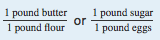are two conversion factors that can be constructed from the pound cake recipe. Other conversion factors are also possible.

3.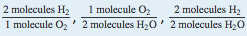and their reciprocals are the conversion factors that can be constructed.

5.

2Na(s) + 2H2O(ℓ) → 2NaOH(aq) + H2(g) and 166 molecules

7.
120 molecules
9.
107 molecules
11.
435,000 molecules
13.

## LicenseStoichiometry by Jessie A. Key is licensed under a Creative Commons Attribution-NonCommercial-ShareAlike 4.0 International License, except where otherwise noted.